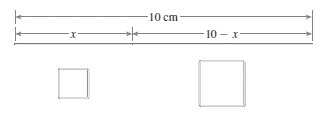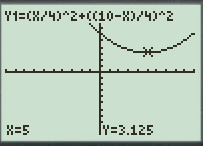# The function that models the total area enclosed by two squares and also the value of x that minimizes the total area of two squares.### Precalculus: Mathematics for Calcu...

6th Edition
Stewart + 5 others
Publisher: Cengage Learning
ISBN: 9780840068071### Precalculus: Mathematics for Calcu...

6th Edition
Stewart + 5 others
Publisher: Cengage Learning
ISBN: 9780840068071

#### Solutions

Chapter 3.1, Problem 74E
To determine

## To find: The function that models the total area enclosed by two squares and also the value of x that minimizes the total area of two squares.

Expert Solution

The function that models the total area enclosed by two squares is equal to A=(x4)2+(10x4)2 and also the value of x that minimizes the total area of two squares is x=5 .

### Explanation of Solution

Given information:

A wire 10cm long is cut into two pieces, one of length x and other of length 10x , as shown in figure below. Each piece is bent in shape of a square.Calculation:

As given the length of one wire is x and the wire is bent in shape of square. So, the length of each side of square is x4 .

As given the length of other wire is 10x and the wire is bent in shape of square. So, the length of each side of second square is 10x4 .

The area of the first square is equal to (x4)2 and the area of the second square is equal to (10x4)2 . The total area of the squares is equal to A=(x4)2+(10x4)2 .

So, the function of the total area of the squares is given by g(x)=(x4)2+(10x4)2 . To minimize the total area of squares graph the function g(x)=(x4)2+(10x4)2 .

To graph a function y=(x4)2+(10x4)2 , follow the steps using graphing calculator.

First press “ON” button on graphical calculator, press Y= key and enter right hand side of the equation y=(x4)2+(10x4)2 after the symbol Y1 . Enter the keystrokes (X÷4)^2+((10X)÷4)^2 .

The display will show the equation,

Y1=(X/4)^2+((10X)/4)^2

Now, press the GRAPH key and TRACE key to produce the graph of given function in standard window as shown in Figure (1).Figure (1)

As observed from the graph, the area function has minimum value at x=5 .

Therefore, the function that models the total area enclosed by two squares is equal to A=(x4)2+(10x4)2 and also the value of x that minimizes the total area of two squares is x=5 .

### Have a homework question?

Subscribe to bartleby learn! Ask subject matter experts 30 homework questions each month. Plus, you’ll have access to millions of step-by-step textbook answers!